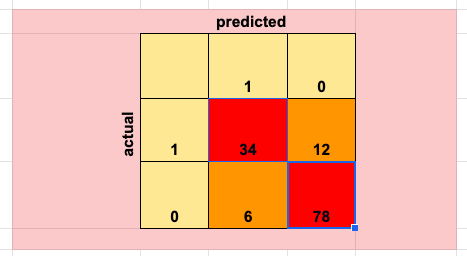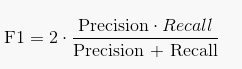Search
•Magdalena Konkiewicz

# Reading a confusion matrixImage from https://pixabay.com

This article will try to explain the above terms and demonstrate how to read a confusion matrix so you can get the most information from it.

What is a confusion matrix?

A confusion matrix is a method of visualizing classification results. You had built a classification model that predicts some values on the test set and you also have some actual values for your target variable to compare with. Confusion matrix will show you if your predictions match the reality and how do they math in more detail.

The confusion matrix below shows predicted versus actual values and gives names to classification pairs: true positives, true negatives, false negatives, and false positives. We will discuss the meaning of each taking a particular example of predicting high blood pressure within patients.True Positives

We will now consider the dataset of 130 patients where based on some feature set we are trying to predict if the patients have high blood pressure or not. In the data set, there are 46 patients that have high blood pressure and the remaining 84 do not. We have run our classification algorithm and we have plotted the predicted values by our model against actual ones. The confusion matrix is shown below. We can see that we are getting 34 true positives. This corresponds to patients that have high blood pressure and our model identified them correctly.True Negatives

We can see that we also get 78 true negative pairs. This means that our algorithm has discovered correctly 78 patients that do not have high blood pressure (they do not have high blood pressure and the model also predicted that they do not). True negatives together with true positives are the parts our model is doing right. We would like to find models that maximize both of them.False Positives

Now we are starting to look at parts that our model is not doing that well. False positives represent the number of patients that our model classified as ones that have high blood pressure but in reality, they do not. Possibly not a critical mistake for the patient but may be costly for the hospital institution who may be treating the patient for the disease he does not have. False positives are also known as Type 1 error.False Negatives

False negatives represent patients that have high blood pressure but our model predicted that they do not. It is also known at Type 2 error and the implication of such a mistake may be different from Type 1 error. In our case, consequences could be even critical as those patients who suffer from high blood pressure would be diagnosed as healthy and not get treatment.Accuracy

A measure that accumulates the correct predictions and puts this number in the contrast of all instances is accuracy. It sums up true positives and true negatives and divides by the number of all instances.The actual formula for accuracy is as below:As you can see we sum all true values (true positives and true negatives) and divide by all examples. In our example, this is (34+78)/130. We get an accuracy of just a bit more than 0.86.

It is important to note that there are some examples where the accuracy on its own could be misleading. So in our example, there are 84 patients that do not have high blood pressure and therefore a model that predicts only not high blood pressure for all patients would gain 64% accuracy (84/130=0.64). Therefore we would like to use other metrics to help model evaluation.

Precision

This is a very important concept. This is the number of patients that have high blood pressure and the model identified correctly (true positives) divided by all patients that our model predicts to have high blood pressure(true positives and false positives). In other words, it measures what is a fraction of correctly identified instances in positive predictions of our model.Recall

Recall represents correctly identified patients who have high blood pressure (true positives) divided by the total number of patients who have the disease (true positives and false negatives). In other words, it measures what is a fraction of all patients that have a disease that our model identifies correctly.If you are still confused you can think of recall as the percentage of total relevant results correctly classified by a model and precision as the percentage of your results that are relevant.

F-score

Precision and recall can be combined into one single metric called f-score. In case you will be giving equal importance to recall and precision you could use it and optimize your model for it. This could be a good compromise if you do not have a strong emphasis if recall or precision is more important. The formula is presented below and it combines both of the metrics we have discussed.Conclusion

In this article, I have introduced a confusion matrix and presented metrics that can be derived from it. While evaluating a classification problem it is important to look at all the metrics mentioned above. However, when trying to optimize or choose the best model increasing one metric may cause the other one to fall. It is important to choose the tradeoff based on model application and understand the implications of these decisions.